NCERT Solutions for Class 7 Maths Chapter 1 Integers Ex 1.1 are part of NCERT Solutions for Class 7 Maths. Here we have given NCERT Solutions for Class 7 Maths Chapter 1 Integers Ex 1.1.

 Board CBSE Textbook NCERT Class Class 7 Subject Maths Chapter Chapter 1 Chapter Name Integers Exercise Ex 1.1 Number of Questions Solved 10 Category NCERT Solutions

## NCERT Solutions for Class 7 Maths Chapter 1 Integers Ex 1.1

Question 1.
Following number line shows the temperature in degree Celsius (°C) at different places on a particular day: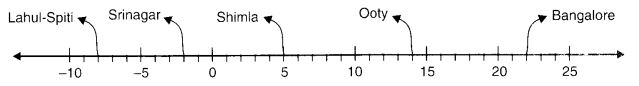(a) Observe this number line and write the temperature of the places marked on it.
(b) What is the temperature difference between the hottest and the coldest places among the above?
(c) What is the temperature difference between Lahul-Spiti and Srinagar?
(d) Can we say temperature of Srinagar and Shimla taken together is less than the temperature at Shimla? Is it also less than the temperature at Srinagar?
Solution:
(a)(b) The hottest place is Bangalore (22 °C) and the coldest place is Lahulspiti (-8°C). The temperature difference between the hottest and the coldest places
= 22 °C – (-8 °C)
= 22 °C + 8 °C
= 30 °C

(c) Temperature difference between Lahul-Spiti and Srinagar
= Temperature of Srinagar
– Temperature of Lahul-Spiti
= – 2°C – (- 8°C)
= – 2°C + 8°C
= 6°C

(d) Yes, we can say that the temperature of Srinagar and Shimla took together is less than the temperature at Shimla as -2 + 5 = 3 and 3 < 5.
This temperature is not less than the temperature at Srinagar.

Question 2.
In a quiz, positive marks are given for correct answers and negative marks are given for incorrect answers. If Jack’s scores in five successive rounds were 25, -5, -10, 15 and 10, what was his total at the end?
Solution:
Total at the end
= 25 + (- 5) + (- 10) + 15 + 10
= (25 + 15 + 10) + {(- 5) + (- 10))
= 50 + (- 15) = 35

Question 3.
At Srinagar, the temperature was – 5°C on Monday and then it dropped by 2°C on Tues¬day. What was the temperature of Srinagar on Tuesday? On Wednesday, it rose by 4°C. What was the temperature on this day?
Solution:
At Srinagar, the temperature was on Monday = -5 °C
Since the temperature was dropped by 2 °C on Tuesday, therefore, the temperature was on Tuesday = (-5-2) °C = -7°C
Also, on Wednesday the temperature rose by 4 °C.
∴ Temperature on Wednesday= (-7 + 4) °C = -3 °C

Question 4.
A plane is flying at the height of 5000 m above sea level. At a particular point, it is e×actly above a submarine floating 1200 m below sea level. What is the vertical distance between them?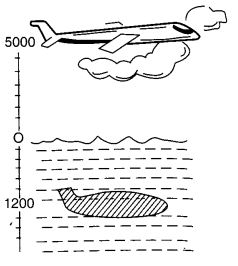Solution:
The vertical distance between the plane and submarine.
= 5000 m – (- 1200 m)
= 5000 m + 1200 m
= 6200 m.

Question 5.
Mohan deposits ₹ 2,000 in his bank account and withdraws ₹1,642 from it, the next day. If the withdrawal of the amount from the account is represented by a negative integer, then how will you represent the amount deposited?
Find the balance in Mohan’s account after the withdrawal.
Solution:
The amount deposited will be represented by a positive integer.
Balance in Mohan’s account after withdrawal
= (+ ₹ 2000) + (- ₹ 1642)
= ₹ (2000-1642)
= ₹ 358

Question 6.
Rita goes 20 km towards the east from point A to point B. From B, she moves 30 km towards the west along the same road. If the distance towards the east is represented by a positive integer then, how will you represent the distance travelled towards the west? By which integer will you represent her final position from A?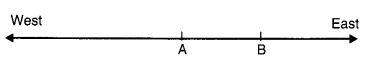Solution:
The distance towards the west = -30 km
Her final position from A
= + 20 km + (- 30) km
= – (30 – 20) km
= – 10 km.

Question 7.
In a magic square each row, column, and diagonal have the same sum. Check which of the following is a magic square.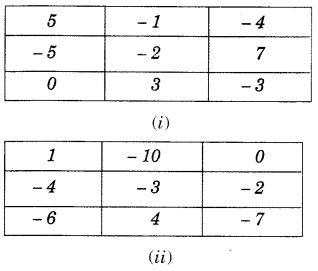Solution:I. Row, Sum = 5 + (- 1) + (- 4)
= 5 + (- 5) = 0
II. Row, Sum = (- 5) + (- 2) + 7
= (- 7) + 7 = 0
III. Row, Sum = 0 + 3 + (- 3)
= 0 + 0 = 0
I. Column, Sum = 5 + (- 5) + 0
= 0 + 0 = 0
II. Column, Sum = (- 1) + (- 2) + 3
= (- 3) + 3 = 0
III. Column, Sum = (- 4) + 7 + (- 3)
= 7 + (- 4) + (- 3)
= 7 + (- 7) = 0
One Diagonal, Sum = 5 + (- 2) + (- 3) = 5 + (- 5) = 0
Other Diagonal, Sum = 0 + (- 2) + (- 4) = 0 + (- 6) = -6 ≠ 0
Therefore, the given square is not a magic square.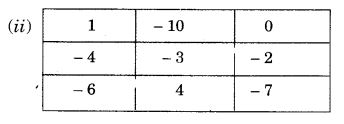I. Row, Sum = 1 + (- 10) + 0 = – 9
II. Row, Sum = (- 4) + (- 3) + (- 2) = – 9
III. Row, Sum = (- 6) + 4 + (- 7)
= 4 + (- 6) + (- 7)
= 4 + (- 13)
= – (13-4) = -9
I. Column, Sum = 1 + (- 4) + (- 6)
= 1 + (- 10)
= -(10 – 1) = – 9
II. Column, Sum = (- 10) + (- 3) + 4
= (-13)+ 4
= – (13-4) = -9
III. Column, Sum = 0 + (- 2) + (- 7)
= 0 + (- 9) = – 9
One Diagonal, Sum = 1 + (- 3) + (- 7)
= 1 + (- 10)
= -(10-1) = -9
Other Diagonal, Sum = (- 6) + (- 3) + 0
= (- 9) + 0 = – 9
Since each row, column, and diagonal ‘ have the same sum, therefore, the given square is a magic square.

Question 8.
Verify a – (- b) = a + b for the following values of a and b.
(i) a = 21, b = 18
(ii) a = 118, b = 125
(iii) a = 75, b = 84
(iv) a = 28, b = 11.
Solution:
(i) a = 21, b = 18
L.H.S. = a – (- b) = 21 – (- 18) = 21 + 18 = 39 …..(1)
R.H.S. = a + b = 21 + 18 = 39 …..(2)
From (1) and (2), we get a -(-b) = a + b

(ii) a = 118, b = 125
L.H.S. = a – (- b) = 118 – (- 125) = 118 + 125 = 243 …(1)
R.H.S. = a + b = 118 + 125 = 243 …(2)
From (1) and (2), we get a -(- b) = a + b

(iii) a = 75, b = 84
L.H.S. = a – (- b) = 75 – (- 84) = 75 + 84 = 159 …(1)
R.H.S. = a + b = 75 + 84 = 159 …(2)
From (1) and (2), we get a – (- b) = a + b

(iv) a = 28, b = 11
L.H.S. = a – (- b) = 28 -(-11) = 28 + 11 = 39 …(1)
R.H.S. = a + b = 28 + 11 = 39 …(2)
From (1) and (2), we get a – (- b) = a + b.

Question 9.
Use the sign of >, < or = in the bo× to make the statements true.
(a) (- 8) + (-4) …… (- 8) – (- 4)
(b) (-3) +7 – (19) …… 15-8 +(-9)
(c) 23 – 41 + 11 …… 23-41- 11
(d) 39 + (-24) – (15) …… 36+ (-52) – (- 36)
(e) – 231 + 79 + 51 …… -399 + 159 + 81.
Solution:
(a) L.H.S. = (- 8) + (- 4)
= – (8 + 4) = – 12
R.H.S. = (- 8) – (- 4)
= – 8 + 4 = – (8 – 4) = – 4
∴ (-8) + (-4) < (-8)-(-4)

(b) L.H.S. = (-3) + 7 – (19)
= + 4 – (19)
= + 4 – 19
= -15
R.H.S. = 15 – 8 + (- 9)
= 7 + (-9) = 7- 9 = -2
∴ (- 3) + 7 – (19) < 15 – 8 + (- 9)

(c) L.H.S. = 23 – 41 + 11
= 23 + 11- 41
= 34 – 41 = – (41 – 34)
= – 7
R.H.S. = 23 – 41 – 11
= 23 – (41 + 11)
= 23 – 52
= – (52 – 23)
= – 29
∴ 23 – 41 + > 23 – 41 – 11

(d) L.H.S. = 39 + (- 24) – (15)
= 39 – 24 – (15)
= 15 – (15) = 0
R.H.S. = 36 + (- 52) – (- 36)
= – (52 – 36) – (- 36)
= – 16 – (- 36)
= – 16 + 36 = 20
∴ 39 + (- 24) – (15) < 36 + (- 52) – (- 36)

(e) L.H.S. = – 231 + 79 + 51
= – 231 + 130
= – (231 – 130) = – 101
R.H.S. = – 399 + 159 + 81
= – 399 + 240
= – (399 – 240)
= – 159
∴ – 231 + 79 + 51 > – 399 + 159 + 81.

Question 10.
A water tank has stepped inside it. A monkey is sitting on the topmost step (i.e., the first step The water level is at the ninth step.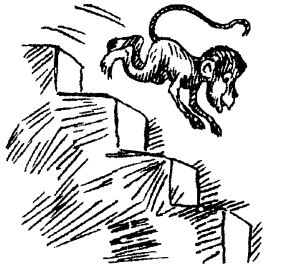(i) He jumps 3 steps down and 2 steps up. In how many jumps will he reach the water level?
(ii) After drinking water he wants to go back. For this, he jumps 4 steps up and then jumps back 2 steps down in every move. In how many jumps will he reach back the top step?
(iii) If the number of steps moved down is represented by negative integers and the number of steps moved up by positive integers, represent his moves in part (i) and (ii) by completing the following;
(a) -3 + 2 – …………. = -8
(b) 4 – 2 + = 8.
In (a) the sum (-8) represents going down by eight steps. So, what will the sum 8 in (b) represent?
Solution:
(i) While going down the monkey jumps 3 steps down and then jumps back 2 steps up. To reach the water level he is to jump as under:
-3+ 2 -3 + 2 – 3 +2 – 3 + 2 – 3 + 2 – 3 = -8 Hence, he takes 11 jumps to reach the water level.
(ii) After drinking water, he jumps back as under to reach the top step as under : 4 – 2+ 4 – 2+ 4 = 8
Hence, he takes 5 jumps to reach back the top.
(iii) (a) – 3 + 2 – 3 + 2 – 3 + 2 – 3 + 2 – 3 + 2 – 3 = -8
(b) 4 – 2 + 4 – 2 + 4 = 8
In (b) the sum 8 represents going up 8 steps.

We hope the NCERT Solutions for Class 7 Maths Chapter 1 Integers Ex 1.1 help you. If you have any query regarding NCERT Solutions for Class 7 Maths Chapter 1 Integers Ex 1.1, drop a comment below and we will get back to you at the earliest.